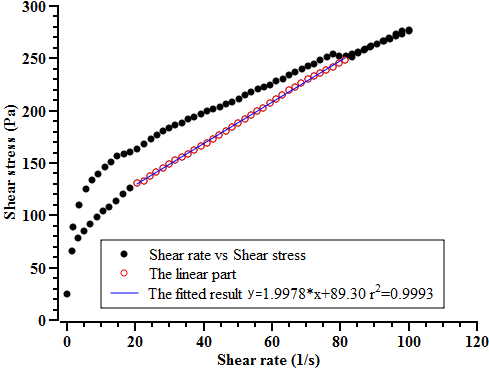Categories

# The method to measure the plastic viscosity of cement paste

In the previous post, I introduced the protocol to measure the yield stress of cement paste. I would like to share the method how to measure the plastic viscosity of cement paste.

The principle determine the plastic viscosity of cement paste is using the relationship between shear rate and shear stress. Applying a serials of shear rate on the cement paste would result in the corresponding shear stress. After obtaining the set of shear rate and shear stress, the plastic viscosity of the paste is calculated by regression.The measured shear rate and shear stress of a cement paste, Measuring device: HAAKE™ VT550 (ViscoTester VT550), CR (Controlled shear Rate): 0-100 1/s; t 120.00 s; T 20.00 °C.

The paste should be measured by increasing shear rate and then the decreasing rate. Typical shear rate applied on cement paste is linearly between 0 and 100 1/s. Since the rheological feature of most of cement pastes is a kind of Bingham model, we can choose the liner part of the decreasing shear curve from the date set (shear rate vs. shear stress) for the regression. The slope from the regression equation is the so-called plastic viscosity.

However, if the rheological cure of the paste behaves rather than Bingham model, selecting an appropriate model is necessary.